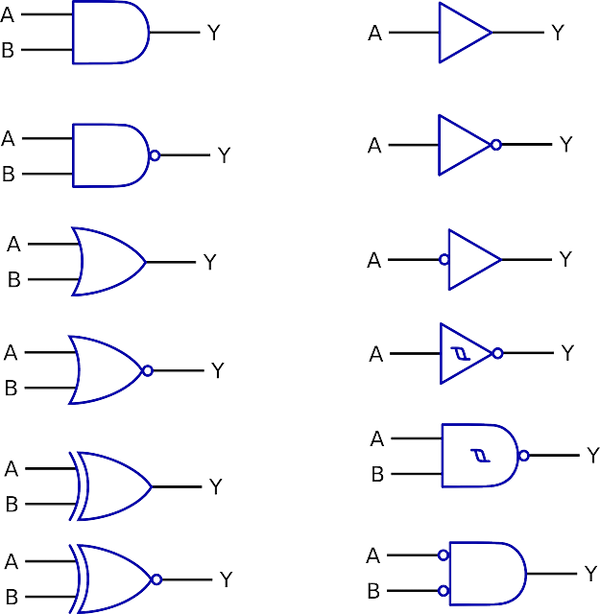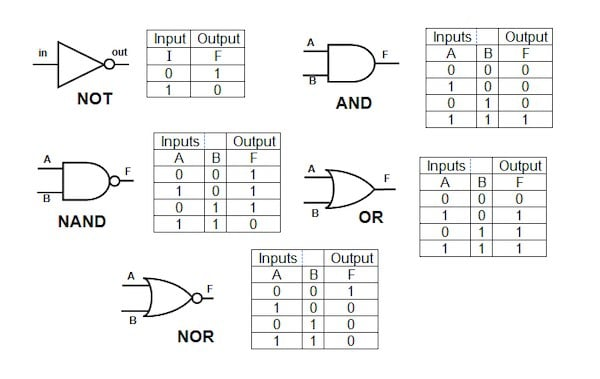# What Are Logic Gates and Why Are They Important?February 24, 2020 by Emmanuel Ikimi
ShareAt some point, even persons with the faintest interest in computer science would have heard about binary code and their functions within computing systems.

As basic as the ones and zeros might seem, binary code is vital to all facets of electronic advancements from simple circuits within everyday home devices to advanced computer chips used to accurately propel rockets into space.

Logic gates are commonly referred to as the fundamental building blocks of digital circuits. Understanding the relationships between the various logic gates allows electronic engineers to design the highly complex systems that enable modern technologies.

### What are Logic Gates?

To efficiently interpret and transmit required digital messages, computing systems need to manipulate the electric current entering and leaving their circuits. This is achieved through the use of devices called logic gates. Logic gates regulate the flow of electricity through an electronic circuit by receiving and modifying binary input and output.

Logic gates are theoretical or practical circuits that make an electronic logic operation possible. In a digital device, logic gates receive single or multiple binary inputs and transform them into single binary output. While the simplest of these devices act independently within transistors, most of them are incorporated into larger electronic systems including arithmetic logic units, multiplexers, and microprocessors.Logic gates are used in computing systems to interpret and transmit required digital messages. (pictured: Logic gate diagrams).

### Types of Logic Gates

There are various examples of logic gates which function differently depending on the type of binary input they receive. Listed below are the most common types of logic gates present in most electronic circuits.

• AND

• OR

• NOT

• NOR

• XOR

• XNOR

• NAND

The activation of each of the above-mentioned logic gate types depends on the presence or absence of a unique binary input which the particular gate is designed to recognize. If the signal corresponds to its configuration, the logic gate gives â€œoneâ€ as its output, if it differs, the output will be recorded in binary code as a â€œzero.â€

#### AND Logic Gates

An AND logic gate is a typical all or none device. To activate this gate, all the inputs reaching it must be ones as well (on). Once a single electric input reaching it is interpreted as zero (off) then the output will be equal zero.

#### OR Logic Gates

This gate type is less restrictive than an AND logic gate. If any of the inputs reaching the gate is a 1 (switched on) then the output will be a binary 1 as well regardless of whether the remaining inputs are ones or zeros.

#### NOT Logic Gates

This type of logic gate is regarded as the contrary type. It operates in the opposite manner to what typically obtains in other types. A NOT logic gate has a single input and output that are opposite to each other at all times. For example, if the input reaching the NOT logic gate is a one then the output will be zero and vice versa.

#### NOR Gates

A NOR gate combines the features of an OR gate with an inverter. The output of this gate type is true (ones) only if both its inputs are false (zeros). Any other combination of inputs will produce a false (zero) output.

#### XNOR Gates

This is another example of a combination logic gate with its distinct features. XNOR gates or exclusive-NOR gates also have an inverter but to achieve a true output, all its binary inputs must be similar regardless of whether they are all true (ones) or false (zeros).

#### NAND Gates

This is a slightly more complex gate type that functions in turn as an AND gate and a NOT gate. The output will be false only if both inputs are true while in any other situation, the output is true.The truth tables and symbols for common logic gates. Image Credit: Schoolphysics.co.uk

### What Are Truth Tables?

Truth tables are a tabular representation of all the possible input and output combinations of each logic gate type. Truth tables provide a quick summary of all potential outputs any distinct gate type can provide.

### What Is a Universal Gate and How Does It Function?

A universal gate is one that can imitate the function of any other gate type. In practice, NAND and NOR gates possess this unique capability. A single NAND gate or a combination of two or more such gates can be made to function either as a NOT gate, an OR gate or an AND gate. Similarly, a NOR gate can be made to act universally by modifying its circuitry to perform all basic gate functions (NOT, OR and AND).

### Key Applications of Logic Gates

Although the theoretical aspects of logic gates may seem a bit abstract, they have very practical real-world applications in both industrial and domestic situations. Logic gates are present in various electronic devices from street lamps to security alarms and ventilation systems. Also, specific gate types are capable of the unique functions outlined below.

#### OR Gate Applications

Many industrial plant setups utilize OR logic gates in carrying out their processes. In most operations, they act as fail-safes for shutting down or activating machinery features that protect them from damage in adverse working conditions. OR gates receive input from temperature and pressure sensors and provide an output that corrects unwanted variations.

#### AND Gate Applications

These gate types function as sentinels screening data flowing through an electronic channel. AND gate can either act as â€œenableâ€ gates allowing the flow of data through a channel or a â€œinhibitâ€ gate preventing data flow. A practical example is the measurement of the frequency of a pulsed wave.

#### NOT Gate Applications

Not gates are simply called inverters because they exert an output directly opposite to the input they receive. A good application that utilizes NOT gates are the wave oscillators used to create clock signals. NOT gates are preferred in these devices as they provide more efficient energy use than other logic gate types.